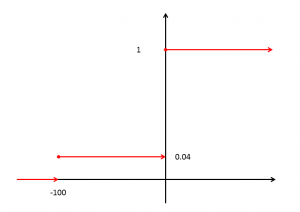24
Apr 18

## Solution to Question 1 from UoL exam 2016, Zone B## Solution to Question 1 from UoL exam 2016, Zone B

This problem is a good preparation for Question 2 from UoL exam 2015, Zone A (FN3142), which is more difficult.

## Problem statement

Two corporations each have a 4% chance of going bankrupt and the event that one of the two companies will go bankrupt is independent of the event that the other company will go bankrupt. Each company has outstanding bonds. A bond from any of the two companies will return$R=0$% if the corporation does not go bankrupt, and if it does, a bondholder will lose the face value of the investment, i.e.,$R=-100$%. Suppose an investor buys $1000 worth of bonds of the first corporation, which is then called portfolio$P_1$, and similarly, an investor buys$1000 worth of bonds of the second corporation, which is then called portfolio$P_2$.
(a) [40 marks] Calculate the VaR at$\alpha=5$% critical level for each portfolio and for the joint portfolio$P=P_1+P_2$.
(b) [30 marks] Is VaR sub-additive in this example? Explain why the absence of sub-additivity may be a concern for risk managers.
(c) [30 marks] The expected shortfall$ES^\alpha$ at the$\alpha=5$% critical level can be defined as$ES^\alpha=E_t[R|R\le VaR^\alpha_{t+1}]$. Calculate the expected shortfall for the portfolios$P_1,\ P_2$ and$P$. Is this risk measure sub-additive?

## Solution

There are a couple of general ideas to understand before embarking on calculations. The return on the bond of one company is a binary variable taking values 0% and -100%. All calculations involving it are similar to the ones for the coin. After doing calculations the return figures can be translated to dollar amounts by multiplying by $1000. While the use of the notions of the distribution function and generalized inverse can be avoided, I prefer to use them to show the general approach. (a) The return on one bond is described by the table Table 1. Probability table for return on one bond  Return values Probability 0 0.96 -100 0.04 Therefore its distribution function can be found in the same way as for the coin:Figure 1. Distribution function for return on one bond The distribution function is shown in red. It is zero for$R<-100$, 0.04 for$-100\le R<0$ and 1 for$0\le R<\infty$. The definition of the VaR requires inversion of this function. The graph of this function has flat pieces and its usual inverse does not exist. We have to use the generalized inverse defined by$F^{-1}(y)=\inf\{x:F(x)\ge y\}$, see the definition of the infimum here. In our case$y=0.05$ and the verbal procedure is: 1) find those returns for which$F(R)\ge 0.05$ (it's the half-axis$[0,\infty)$) and 2) among them find the least return. The answer is$VaR^\alpha=0$%. This is the Value at Risk for each of$P_1,P_2.$ What we do next is very similar to the derivation of the sampling distribution for two coins. Table 2. Joint probability table for returns on two portfolios  First portfolio 0 -100 Second portfolio 0$0.96^2=0.9216$$0.96^2=0.9216$$0.04\times 0.96=0.0384$$0.04\times 0.96=0.0384$ -100$0.04\times 0.96=0.0384$$0.04\times 0.96=0.0384$$0.04^2=0.0016$$0.04^2=0.0016$ The main body of the table contains probabilities of pairs$(R_1,R_2)$ of the two returns. For the total portfolio the possible return values are 0 (none of the companies goes bankrupt), -50 (one goes bankrupt and the other does not) and -100 (both go bankrupt). The corresponding probabilities follow from Table 2 and we get Table 3. Probability table for return on the total portfolio  Total return Probabilities 0 0.9216 -50$2\times 0.0384=0.0768$$2\times 0.0384=0.0768$ -100 0.0016 This table results in the following distribution function:Figure 2. Distribution function for return on two bonds Since 0.0784>0.05, the Value at Risk is -50% (use the generalized inverse). (b) Translating the percentages to dollars, at 5% the risk for each of the bonds is$0 and for the total portfolio it is $1000 (50% of$2000; I am passing from negative percentages to positive loss figures).

We say that Value at Risk is sub-additive if$VaR_P^\alpha\le Var^\alpha_{P_1}+Var^\alpha_{P_2}$. Our calculations show that Value at Risk is not sub-additive in case of independent returns. This has an important practical implication. Suppose that a financial institution has several branches and each of them keeps their Value at Risk, say, at zero. Nevertheless, the Value at Risk for the whole institution may well be large and threaten its stability.

(c) Here we have to apply the definition of the conditional expectation:$ES^\alpha_{P_i}=\frac{E_t[R1_{\{R\le VaR^\alpha_{t+1}\}}]}{P(R\le VaR^\alpha_{t+1})}$. Since$P(R\le 0)=0.96+0.04=1$, this is the same as$ES^\alpha_{P_i}=E_t[R1_{\{R\le 0\}}]=0\times 0.96+(-100)\times 0.04=-4$%,$i=1,2$.

For the total portfolio we get$ES^\alpha_P=\frac{E_t[R1_{\{R\le -50\}}]}{P(R\le -50)}=\frac{(-50)\times 0.0768+(-100)\times 0.0016}{0.0768+0.0016}=\frac{-3.84-0.16}{0.0784}=-51.02$%.

In monetary terms, this translates (again passing to positive values) to $40 for each bond and to$1020.40 for the total portfolio. The conclusion is that expected shortfall is not sub-additive.